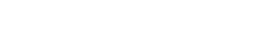# Half integral of x

In my previous post I showed you how to take the half derivative of x. Here, I am going to explain how to find the half integral of x.

We can write the half integral of x as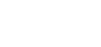This is a way I’ve come up with to represent the half integral of a function, as I couldn’t find anything about it.

We can write the second, third, fourth integral of a function as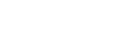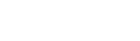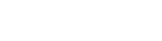and, in general,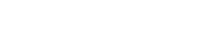or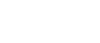So we will write the half integral of x like this: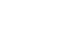Here we found that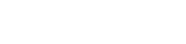And, in particular, that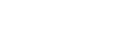This is the half derivative of x, i.e. the inverse of the half integral of x.

We know that the integral of x is x²/2 and that the derivative of x² is 2x. As you can see, the coefficient of the x in the result of the integral is the reciprocal of that of the derivative. This means that the coefficient of the x in result of the half integral of x is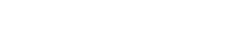What we need to find now is the exponent of the x. We can give a look at these integrals: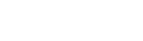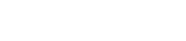As you can see, the exponent of the x in the result is n (the order of the integral) + 1. This means the coefficient of the x for the half integral is 1/2+1=3/2.

Overall,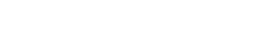Therefore,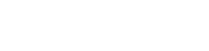In the previous post we saw that (-0.5)! = sqrt(pi), so